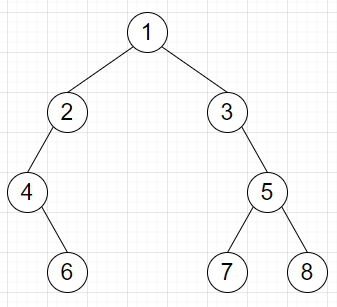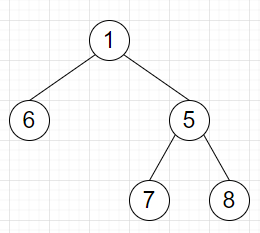# Program to remove all nodes with only one child from a binary tree in Python?

Suppose we have a binary tree root; we have to remove all nodes with only one child.

So, if the input is likethen the output will beTo solve this, we will follow these steps:

• Define a method called solve(), this will take tree root

• if root is null, then

• return root

• if left of root is null and right of root is null, then

• return root

• if left of root is null, then

• return solve(right of root)

• if right of root is null, then

• return solve(left of root)

• left of root := solve(left of root)

• right of root := solve(right of root)

• return root

## Example

class TreeNode:
def __init__(self, data, left = None, right = None):
self.data = data
self.left = left
self.right = right

def print_tree(root):
if root is not None:
print_tree(root.left)
print(root.data, end = ', ')
print_tree(root.right)

class Solution:
def solve(self, root):
if not root:
return root

if not root.left and not root.right:
return root

if not root.left:
return self.solve(root.right)

if not root.right:
return self.solve(root.left)

root.left = self.solve(root.left)
root.right = self.solve(root.right)

return root

ob = Solution()
root = TreeNode(1)
root.left = TreeNode(2)
root.right = TreeNode(3)
root.left.left = TreeNode(4)
root.right.right = TreeNode(5)
root.left.left.right = TreeNode(6)
root.right.right.left = TreeNode(7)
root.right.right.right = TreeNode(8)

res = ob.solve(root)
print_tree(res)

## Input

root = TreeNode(1)
root.left = TreeNode(2)
root.right = TreeNode(3)
root.left.left = TreeNode(4)
root.right.right = TreeNode(5)
root.left.left.right = TreeNode(6)
root.right.right.left = TreeNode(7)
root.right.right.right = TreeNode(8)

## Output

6, 1, 7, 5, 8,